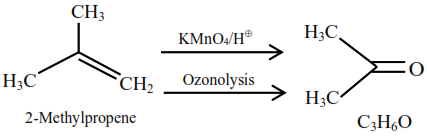# An organic compound`
Question:

An organic compound ' $\mathrm{A}^{\prime} \mathrm{C}_{4} \mathrm{H}_{8}$ on treatment with $\mathrm{KMnO}_{4} / \mathrm{H}^{+}$yields compound ' $\mathrm{B}$ ' $\mathrm{C}_{3} \mathrm{H}_{6} \mathrm{O}$.

Compound 'A' also yields compound 'B' an ozonolysis. Compound 'A' is :

1. 2-Methylpropene

2. 1-Methylcyclopropane

3. But-2-ene

4. Cyclobutane

Correct Option: 1

Solution: